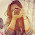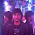## Wednesday, February 5, 2014

### Power Electronics Objective Type Questions: Part-13

Power Electronics Multiple Choice Questions

 During forward blocking state, the SCR has
(a) Low current, medium voltage
(b) Low current, large voltage
(c) Medium current, large voltage
(d) Large current, low voltage

Answer: B
 Once SCR starts conducting a forward current, its gate loses control over
(a) Anode circuit current only
(b) Anode circuit voltage only
(c) Anode circuit voltage and current
(d) Anode circuit voltage, current and time

Answer: C
 In a SCRs
(a) Both Latching current and holding current are associated with turn-off process
(b) Latching current is associated with turn-off process and holding current with turn on process
(c) Holding current is associated with turn-off process and Latching current with turn-on process
(d) Both Latching current and holding current are associated with turn on process

Answer: C
 The SCR can be termed as
(a) DC switch
(b) AC switch
(c) Square-wave switch
(d) Either A or B

Answer: A
 In a thyristor, the magnitude of anode current will
(a) Increase if gate current is increased
(b) Increase if gate current is decreased
(c) Decrease if gate current is decreased
(d) Not change with any variation in gate current

Answer: D
 Turn on time of an SCR can be reduced by using a
(a) Rectangular pulse of high amplitude and narrow width
(b) Rectangular pulse of low amplitude and wide width
(c) Triangular pulse
(d) Trapezoidal pulse

Answer: A
 Turn off time of an SCR in series with RL circuit can be reduced by
(a) Increasing circuit resistance R
(b) Decreasing circuit resistance R
(c) Increasing circuit inductance L
(d) Decreasing circuit inductance L
Options:
1) B, C
2) A, D
3) B, D
4) D only

Answer: 4
 A forward voltage can be applied to an SCR after its
(a) Anode current reduces to zero
(b) Gate recovery time
(c) Reverse recovery time
(d) Anode voltage reduces to zero

Answer: B
 Turn-off time of an SCR is measured from the instant
(a) Anode current becomes zero
(b) Anode voltage becomes zero
(c) Anode voltage and anode current become zero at the same time
(d) Gate current becomes zero

Answer: A
 In an SCR, anode current flows over a narrow region near the gate during
(a) Delay time td
(b) Rise time tr and spread time tp
(c) td and tp
(d) td and tr

Answer: D
 Gate characteristic of a thyristor
(a) Is straight line passing through origin
(b) Is of the type Vg = a + bIg
(c) Is a curve between Vg and Ig
(d) Has a spread between two curves of Vg-Ig

Answer: D
 Surge current rating of an SCR specified the maximum
(a) Repetitive current with sine wave
(b) Non-repetitive current with rectangular wave
(c) Non-repetitive current with sine wave
(d) Repetitive current with rectangular wave

Answer: C
 The di/dt rating of an SCR is specified for its
(a) Decaying anode current
(b) Decaying gate current
(c) Rising gate current
(d) Rising anode current

Answer: D
 For an SCR, dv/dt protection is achieved through the use of
(a) RL in series with SCR
(b) RC across
(c) L in series with
(d) RC in series with

Answer: B
 For an SCR, di/dt protection is achieved through the use of
(a) R in series with SCR
(b) RL in series with SCR
(c) L in series with SCR
(d) L across SCR

Answer: C

Solution Hint:
 SCR will allow current flow in one direction only. So the ideal SCR is similar to DC switch
 By the decreasing the Inductor L value, the di/dt is increasing. ie, fast turn off process
 Snubber circuit ( RC circuit across device) is used for dv/dt protection
 Inductor will oppose sudden change in current (di/dt). For inductor, series resistance is not required. But in snubber circuit, R is mandatory for dissipating the stored energy in the capacitor.
8:23 PM

#### 4 comments:

1.very nice superb work.
we are thankful to u mam.

2.how to subscribe for electronics material to gmail

3.can you explain why once an SCR starts conducting the gate loses control over it? In question no.2?

4.Great Job....The questions are Very good.
Can we know the source of these objective questions are all answers error free.
plz reply